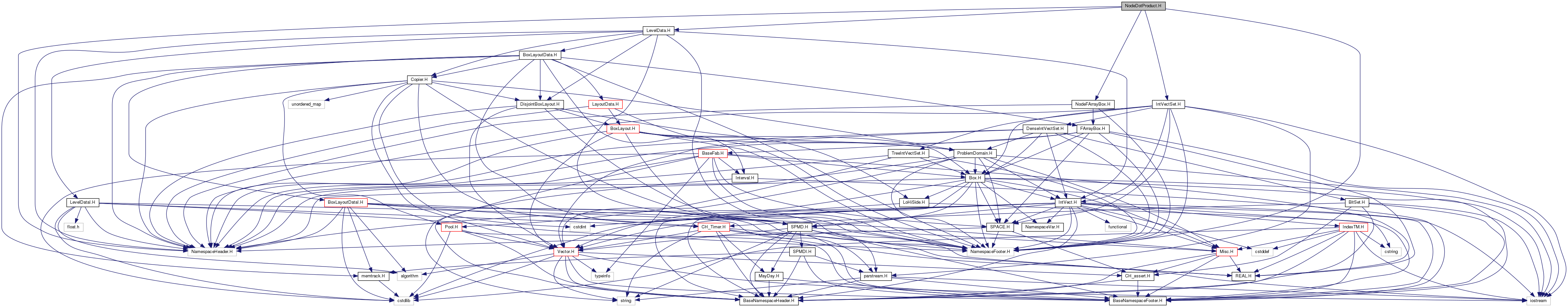Chombo + EB + MF  3.2
NodeDotProduct.H File Reference
`#include "NodeFArrayBox.H"`
`#include "LevelData.H"`
`#include "IntVectSet.H"`
`#include "NamespaceHeader.H"`
`#include "NamespaceFooter.H"`
Include dependency graph for NodeDotProduct.H:Go to the source code of this file.

## Macros

#define _NODEDOTPRODUCT_H_

## Functions

Real DotProductNodes (const BoxLayoutData< NodeFArrayBox > &a_dataOne, const BoxLayoutData< NodeFArrayBox > &a_dataTwo, const BoxLayout &a_dblIn)
Computes dot product of two sets of data on the same set of grids. More...

Real DotProductNodes (const BoxLayoutData< NodeFArrayBox > &a_dataOne, const BoxLayoutData< NodeFArrayBox > &a_dataTwo, const BoxLayout &a_dblIn, const Interval &a_comps)
Computes dot product of two sets of data on the same set of grids. More...

Real DotProductNodes (const LevelData< NodeFArrayBox > &a_dataOne, const LevelData< NodeFArrayBox > &a_dataTwo, const ProblemDomain &a_domain, const LayoutData< Vector< IntVectSet > > &a_IVSVext, const Interval &a_comps)
Computes dot product of two sets of data on the same set of grids. More...

Real DotProductNodes (const LevelData< NodeFArrayBox > &a_dataOne, const LevelData< NodeFArrayBox > &a_dataTwo, const Box &a_domain, const LayoutData< Vector< IntVectSet > > &a_IVSVext, const Interval &a_comps)
Computes dot product of two sets of data on the same set of grids. More...

Real DotProductNodes (const LevelData< NodeFArrayBox > &a_dataOne, const LevelData< NodeFArrayBox > &a_dataTwo, const ProblemDomain &a_domain, const Interval &a_comps)
Computes dot product of two sets of data on the same set of grids. More...

Real DotProductNodes (const LevelData< NodeFArrayBox > &a_dataOne, const LevelData< NodeFArrayBox > &a_dataTwo, const Box &a_domain, const Interval &a_comps)
Computes dot product of two sets of data on the same set of grids. More...

## ◆ _NODEDOTPRODUCT_H_

 #define _NODEDOTPRODUCT_H_

## ◆ DotProductNodes() [1/6]

 Real DotProductNodes ( const BoxLayoutData< NodeFArrayBox > & a_dataOne, const BoxLayoutData< NodeFArrayBox > & a_dataTwo, const BoxLayout & a_dblIn )

Computes dot product of two sets of data on the same set of grids.

The dot product computed by this function is the sum over all components and all points of the product of a_dataOne and a_dataTwo, weighted by trapezoidal integration rule weights.

Parameters
 a_dataOne first set of data a_dataTwo second set of data a_dblIn CELL-centered layout, on the nodes of which we calculate the dot product

## ◆ DotProductNodes() [2/6]

 Real DotProductNodes ( const BoxLayoutData< NodeFArrayBox > & a_dataOne, const BoxLayoutData< NodeFArrayBox > & a_dataTwo, const BoxLayout & a_dblIn, const Interval & a_comps )

Computes dot product of two sets of data on the same set of grids.

The dot product computed by this function is the sum over the components in a_comps and over all points of the product of a_dataOne and a_dataTwo, weighted by trapezoidal integration rule weights.

Parameters
 a_dataOne first set of data a_dataTwo second set of data a_dblIn CELL-centered layout, on the nodes of which we calculate the dot product a_comps components over which to take sum

## ◆ DotProductNodes() [3/6]

 Real DotProductNodes ( const LevelData< NodeFArrayBox > & a_dataOne, const LevelData< NodeFArrayBox > & a_dataTwo, const ProblemDomain & a_domain, const LayoutData< Vector< IntVectSet > > & a_IVSVext, const Interval & a_comps )

Computes dot product of two sets of data on the same set of grids.

The dot product computed by this function is the sum over the components in a_comps and over all VALID nodes of the product of a_dataOne and a_dataTwo.

The a_IVSVext argument is obtained from the calls:
``` interiorBoundaryNodes(IVSVint, a_dataOne.getBoxes(), a_domain); exteriorBoundaryNodes(a_IVSVext, IVSVint, a_dataOne.getBoxes(); ```

Parameters
 a_dataOne first set of data a_dataTwo second set of data a_domain physical domain a_IVSVext external boundary nodes a_comps components over which to take sum

## ◆ DotProductNodes() [4/6]

 Real DotProductNodes ( const LevelData< NodeFArrayBox > & a_dataOne, const LevelData< NodeFArrayBox > & a_dataTwo, const Box & a_domain, const LayoutData< Vector< IntVectSet > > & a_IVSVext, const Interval & a_comps )

Computes dot product of two sets of data on the same set of grids.

The dot product computed by this function is the sum over the components in a_comps and over all VALID nodes of the product of a_dataOne and a_dataTwo.

The a_IVSVext argument is obtained from the calls:
``` interiorBoundaryNodes(IVSVint, a_dataOne.getBoxes(), a_domain); exteriorBoundaryNodes(a_IVSVext, IVSVint, a_dataOne.getBoxes(); ```

Parameters
 a_dataOne first set of data a_dataTwo second set of data a_domain physical domain a_IVSVext external boundary nodes a_comps components over which to take sum

## ◆ DotProductNodes() [5/6]

 Real DotProductNodes ( const LevelData< NodeFArrayBox > & a_dataOne, const LevelData< NodeFArrayBox > & a_dataTwo, const ProblemDomain & a_domain, const Interval & a_comps )

Computes dot product of two sets of data on the same set of grids.

The dot product computed by this function is the sum over all components and all points of the product of a_dataOne and a_dataTwo, weighted by trapezoidal integration rule weights.

This function computes exterior boundary nodes. It is more efficient to precompute these boundary-node objects and call the function that takes a_IVSVext as an argument.

Parameters
 a_dataOne first set of data a_dataTwo second set of data a_domain physical domain a_comps components over which to take sum

## ◆ DotProductNodes() [6/6]

 Real DotProductNodes ( const LevelData< NodeFArrayBox > & a_dataOne, const LevelData< NodeFArrayBox > & a_dataTwo, const Box & a_domain, const Interval & a_comps )

Computes dot product of two sets of data on the same set of grids.

The dot product computed by this function is the sum over all components and all points of the product of a_dataOne and a_dataTwo, weighted by trapezoidal integration rule weights.

This function computes exterior boundary nodes. It is more efficient to precompute these boundary-node objects and call the function that takes a_IVSVext as an argument.

Parameters
 a_dataOne first set of data a_dataTwo second set of data a_domain physical domain a_comps components over which to take sum